×

×

KSU / Chemistry / CHEM 10060 / What are the five main points that make up dalton's atomic theory?

# What are the five main points that make up dalton's atomic theory? Description

EXAM 1 STUDY GUIDE

## What are the 5 main points of dalton's atomic theory?Dalton’s Atomic Theory

•All matter consists of indivisible atoms

• Atoms of one kind of element are identical in mass and  properties; atoms of different kinds of elements are  different

•Compounds are made up of definite numbers of atoms of  the component elements

•The weight of a compound equals the sum of the weights  of the component elements

Rutherford’s conducted further experiments which  contradicted Thompson’s model.

To explain his results Rutherford postulated

Most of the mass of the atom and all its positive charge  was located in a concentrated core, called the nucleus.  Most of the total volume of the atom is empty in which  electrons move around the positive core.

## What limits the “boundary” of a molecule?Model of the Atom

Since the times of Rutherford, many more subatomic  particles have been discovered.

However, for chemists three sub-atomic particles are all that  we need to focus on – ELECTRON, PROTON, NEUTRON.

Electrons are –1, protons +1 and neutrons are neutral.

Atoms have an equal number of electrons and protons they  are electrically neutral.

Protons and neutrons make up the heavy, positive core, the NUCLEUS which  occupies a small volume of the atom. We also discuss several other topics like When does learning confer a fitness advantage?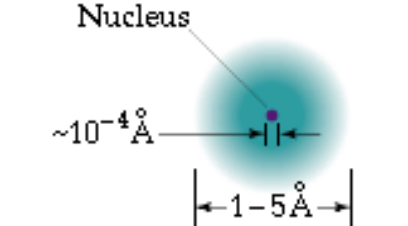## How many grams of water are there in 0.2000 moles of water?Atoms of the SAME element can have different number of  NEUTRONS.

These atoms of the SAME elements but with different  number of neutrons are called ISOTOPES.

Hence isotopes of the same elements have the same  number of protons and electrons, but different number of  neutrons and hence different masses.

EXAMPLE – Carbon has three isotopes C12, C13, C14. Each  of these isotopes differ by the number of neutrons – ALL  have SIX protons. C12 has SIX neutron, C13 has SEVEN and  C14 has EIGHT.

To denote the number of protons and neutrons in an atom  the following symbol notation is used

126C where 12 denotes SUM OF PROTONS + NEUTRONS  6 denotes the number of PROTONS

So for the isotopes of carbon the complete chemical  symbols are: 126C, 136C, 146C

The superscript, which is the sum of the number of  protons and neutrons, is called the MASS NUMBER (A). We also discuss several other topics like What happened during the tokugawa period?

The subscript indicates the number of protons and is called  the ATOMIC NUMBER (Z) .

How many protons, neutrons, and electrons are there in

Au 197

79

197 is the mass number and refers to the total number of protons and neutrons. 79 is the  atomic number and refers to the number of protons. Hence this atom has 79 electrons and  197-79 = 118 neutrons

Average Relative Atomic Mass

Because the abundance of the isotopes of different  elements are essentially constant, we can define an  AVERAGE RELATIVE ATOMIC mass

Average Relative Atomic Mass = average mass of atoms of  an element =

(Abundance)A(Mass)A + (Abundance)B(Mass)A + …

Problem

Naturally occurring chlorine has two isotopes, 3517Cl, 3717Cl.  The 35-Cl isotope has a relative atomic mass of 34.9688  and an abundance of 75.77% and the 37-Cl isotope has a  relative atomic mass of 36.9659 and an abundance of  24.23%. Calculate the average atomic mass of Cl. We also discuss several other topics like What causes panic disorder?

Average Atomic Mass of Cl = (0.7577x34.9659) +  (.2423x36.9659)

= 35.4527

Average relative atomic mass of C is 12.0107 accounting for 12C (98.892%, relative atomic  mass 12.000000) and 13C (1.108%, relative atomic mass 13.003354)We also discuss several other topics like What does it mean to be jointly and severally liable?

PROBLEM

How many moles of Fe are there in 8.232 g of Fe? How many atoms are there in 8.232 g of Fe?

Moles of Fe = 8.232 g Fe x 1 mole = 0.1474 mol Fe 55.85 g Fe

How many grams of water are there in 0.2000 moles of water?

0.2000 mol H2O x 18.015 g H2O = 3.603 g H2O 1 mol H2O

What limits the “boundary” of a molecule?

Atoms in molecules are held together by strong interactions  called CHEMICAL BONDS

Interactions between neutral atoms in a molecule (e.g. H2,  H2O) is called a COVALENT bond, forming covalent compounds

Interactions between charged elements (IONS) result in a different kind of chemical bond,  called an IONIC BOND Don't forget about the age old question of What is the function of the interneurons?

Compounds formed via interactions between ionic  (charged) elements are called ionic compounds.

ELEMENTAL ANALYSIS

Determination of the relative amounts of the elements in a  compound

Determines the EMPIRICAL FORMULA which is the simplest  possible formula and indicates the relative amounts of  constituent elements

For example, the molecular formula of hydrogen peroxide is  H2O2; its empirical formula is HO.

The empirical and the chemical formula can be the same, for  example, H2O.

For ionic compounds, the empirical formula is the same as  the chemical formula (NaCl)

Problem If you want to learn more check out What are the main sources of heat?

An oxide of nitrogen is analyzed and found to contain 25.9%  N and 74.1% O. What is the empirical formula of the  compound?

In 100.0 g of compound:

# moles of N = 25.9 g N / (14.01 g/mol) = 1.85 mol # moles of O = 74.1 g O / (16.00 g/mol) = 4.63 mol

Ratio of O: N :: 2.5:1

Must be whole numbers – N2O5

Determining Chemical Formulas

The empirical formula tells you the simplest ratio of the  individual elements in the compound.

For an ionic compound this information is enough.

For a molecular compound this may not be enough since the  empirical formula may not be the molecular formula.

Knowledge of the MOLAR MASS of the compound and its  empirical formula, allows the molecular formula to be  determined.

Elemental analysis of a sugar shows that it consists of 40.0%  carbon (C), 6.7% hydrogen (H), 53.3% oxygen (O). The molar  mass of the compound was found to be 180.0 g/mol. What is  the molecular formula of the compound?

moles of C in 100.0 g of compound

= (mass of C g)/(atomic mass g/mol)

= 40.0/12.01 = 3.33 mol C in 100.0 g of compound

moles of H in 100.0 g of compound

= (mass of H g)/(atomic mass g/mol)

= 6.7/1.01 = 6.7 mol H in 100.0 g of compound

moles of O in 100.0 g of compound

= (mass of O g)/(atomic mass g/mol)

= 53.3/16.00 = 3.33 mol O in 100.0 g of compound Ratio of C:H:O :: 1:2:1; hence empirical formula is CH2O

Molar mass of empirical formula = 12.01 + 2(1.01) + 16.00 =  30.0 g/mol

Ratio of molar mass of compound : molar mass of empirical  formula

180.0/30.0 = 6.0

molecular formula is (CH2O) 6 or C6H12O6

An important aspect of a chemical reaction is that MASS IS  ALWAYS CONSERVED - i.e. the total mass of the reactants  must equal the total mass of the products.

To ensure that mass is conserved, we have to keep track of  the number of atoms of each element in the reactants and  number of atoms of each element in the products

Writing Chemical Equations H2 + O2 ???? H2O

reactants

products

Mass has not been conserved - equation is not BALANCED

Need to make sure that the number of atoms of a given element is the same on either side.

H2 + O2 ???? H2O

H is balanced, but O is not.

To balance O, multiply H2O by 2

H2 + O2 ???? 2 H2O

Now O is balanced but not H (4 H’s on right, 2 on left) Multiply H2 by 2

2H2 + O2 ???? 2 H2O

Chemical Stoichiometry

Reactants are consumed and products are formed in  definite proportions.

These proportions are given by the coefficients in the  balanced equations for chemical reactions

The calculation of the quantities of reactants and products  is called STOICHIOMETRY.

Stoichiometry is the use of chemical equations to calculate  quantities of substances that take part in chemical  reactions.

To do a stoichiometric calculation, the chemical equation  for the calculation must be balanced.

Equations are read in terms of moles of reactants and  product

2H2(g) + O2(g) ???? 2H2O(g)

2 moles of H2(g) reacts with 1 mole of O2(g) to form 2  moles of H2O(g)

Or:

2No molecules of H2(g) + No molecules of O2(g) ???? 2No molecules of H2O(g)

Problem

Consider the reaction of 100 g of H2(g) with sufficient O2(g)  to produce the stoichiometric quantity of H2O(g).  (stoichiometric quantities are the exact amounts of  reactants and products predicted by balanced equations).  Calculate the mass of H2O formed.

Need a balanced equation for this reaction

2H2(g) + O2(g) ???? 2H2O(g)

To find the mass of water formed, need to find the number of moles of H2that reacted:

(mass of H2) = (100.g H2)/(2.02 g/mol) = 49.5 mol H2

(molar mass of H2)

2 moles of H2reacts with 1 mole of O2to form 2 moles of H2O => 49.5 moles of H2 will form 49.5 moles of H2O

Hence, the mass of H2O(g) formed

=(49.5 moles H2O) x (18.02 g/mol) = 892 g H2O

Problem:

Calcium carbonate CaCO3(s) is decomposed by HCl(aq) to give CaCl2 (aq), CO2(g) and H2O(l). If 10.0g of CaCO3 are treated with 10.0 g of HCl,  how many grams of CO2 are generated?

First write a balanced equation for the reaction:

CaCO3(s) + 2HCl(aq) ???? CaCl2(aq) + H2O(l) + CO2(g)

1 mole of CaCO3reacts with 2 moles of HCl to form 1 mole of CO2

Moles of CaCO3 = (10.0 g)/(100.0g/mol) = 0.100 mole CaCO3 Moles of HCl = (10.0 g)/(36.5 g/mol) = 0.274 mol HCl

Hence 0.1 mole of CaCO3reacts with 0.2 moles of HCl.  Since the amount of HCl present is greater that 0.2, HCl is  in excess and CaCO3is the limiting reactant.

Hence, 0.1 mole of CO2formed.

Mass of CO2formed = 0.1 mol x 44.01 g/mol = 4.40 g CO2

Product Yields

In the previous calculation 4.40 g is the amount of CO2 we  would expect to be formed

4.40 g CO2is the CALCULATED or the THEORETICAL  PRODUCT YIELD.

This assumes that the reaction goes to completion, and that  there are no competing factors that may reduce the amount  of CO2formed.

The measured amount of product formed is called the  ACTUAL YIELD which is often smaller than the theoretical  yield.

Percentage Yield = (actual yield) x 100 %

(theoretical yield)

The larger the % yield the more cost effective is the process and hence a more likely  candidate for industrial scale processes

(other factors are also important: the nature of the by-products - are they environmentally  safe -, the cost of the starting materials, etc.)

Chemical Bonding

A chemical bond results from strong electrostatic  interactions between two atoms.

The nature of the atoms determines the kind of bond.

COVALENT bonds result from a strong interaction between  NEUTRAL atoms

Each atom donates an electron resulting in a pair of electrons  that are SHARED between the two atoms

For example, consider a hydrogen molecule, H2. When the  two hydrogen, H, atoms are far apart from each other they  do not feel any interaction.

As they come closer each “feels” the presence of the other.

The electron on each H atom occupies a volume that covers  both H atoms and a COVALENT bond is formed.

Once the bond has been formed, the two electrons are  shared by BOTH H atoms.

An electron density plot for the H2 molecule shows that the  shared electrons occupy a volume equally distributed over  BOTH H atoms.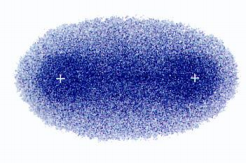Electron Density for the H2 molecule

What factors determine if an atom forms a covalent or ionic  bond with another atom?

The number of electrons in an atom, particularly the number  of the electrons furthest away from the nucleus determines  the atom’s reactivity and hence its tendency to form covalent  or ionic bonds.

These outermost electrons are the one’s that are more likely  to “feel” the presence of other atoms and hence the one’s  involved in bonding i.e. in reactions.

Chemistry of an element depends almost entirely on the  number of electrons, and hence its atomic number.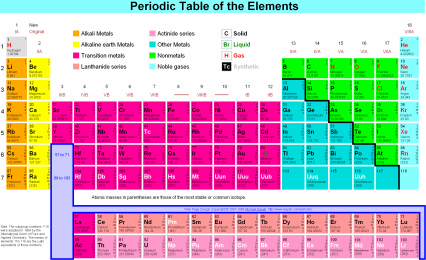Columns are called GROUPS (FAMILIES) and rows are called  PERIODS.

Elements in a group have similar chemical and physical  properties.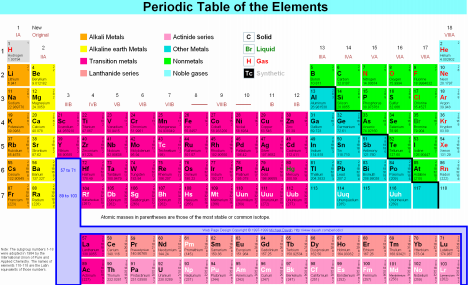The total number of electrons within a group is different,  increasing in number down a group

However, the number of electrons furthest away from the  nucleus, called the OUTER or VALENCE electrons is the  same for all elements in a group.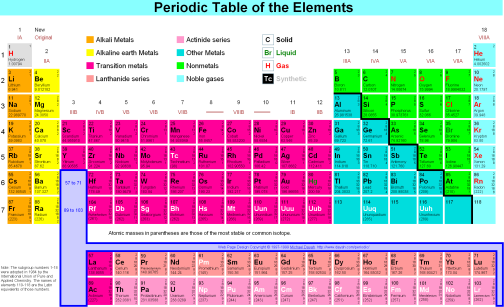Groups are referred to by names, which often derive from  their properties

I – Alkali metals; II – Alkaline Earth metals

VII – Halogens; VIII – Noble gases

The elements in the middle block are called TRANSITION  ELEMENTS

ELECTRONEGATIVITY measures the tendency of one atom to  attract electrons from another atom to which it is bonded.

For example, Metallic elements loose electrons (to form positive  ions) more readily than non-metallic elements

Metallic elements are hence referred to as being more  ELECTROPOSITIVE that non-metals.

Non-metals are more ELECTRONEGATIVE compared to metals

Based on the position of elements in the periodic table, we  can determine the kind of bond formed

Generally:

Nonmetallic element + nonmetallic element ???? Molecular  compound

Molecular compounds are typically gases, liquids, or low  melting point solids and are characteristically poor  conductors. Examples are H2O, CH4, NH3.

TABLE

Generally,

Metallic compound + nonmetallic compound ???? IONIC  compound

Ionic compounds are generally high-melting solids that are  good conductors of heat and electricity in the molten state.

Examples are NaCl, common salt, and NaF, sodium fluoride. TABLE

NAMING COMPOUNDS

The chemical formula represents the composition of each  molecule.

In writing the chemical formula, in almost all cases the  element farthest to the left of the periodic table is written  first.

So for example the chemical formula of a compound that  contains one sulfur atom and six fluorine atoms is SF6.

If the two elements are in the same period, the symbol of the  element of that is lower in the group (i.e. heavier) is written  first e.g. IF3.

In naming covalent compounds, the name of the first element  in the formula is unchanged.

The suffix “-ide” is added to the second element.

Often a prefix to the name of the second element indicates the  number of the element in the compound

SF6 – sulfur hexafluoride

P4O10 – tetraphosphorous decoxide

CO – carbon monoxide

CO2 – carbon dioxide

Electrolytes & nonelectrolytes

Na+

battery +

+

bulb

-

Cl

NaCl(s) --> Na+(aq) + Cl-(aq)

deionized water + NaCl

When the battery is turned on the Na+ions flow toward the negative plate (anode) and  the Cl ions to the positive plate (cathode).

The flow of ions constitutes a current. The circuit is now  complete, current flows through the circuit, and the bulb  turns on.

NaCl is called an electrolyte.

Electrolyte : a compound which when dissolved in a solvent  dissociates to form ions in solution.

Typically electrolytes are ionic compounds since they  dissolve in solution to form ions.

Example: K2SO4

Some covalent compounds (like acids and bases) can  dissociate in solution to form ions.

Electrolytes are characterized as being strong or weak.

The strength of an electrolyte depends on the degree to  which the compound dissociates in water to form ions.

Hence ionic compounds like NaCl and K2SO4 which  dissociate completely in water are strong electrolytes.

Weak electrolytes do not dissociate extensively in water consequently the conductance of a solution of a weak  electrolyte in low.

Non-electrolytes do not dissociate in solution to form ions  and hence their solutions do not conduct electricity.

Page ExpiredIt looks like your free minutes have expired! Lucky for you we have all the content you need, just sign up here